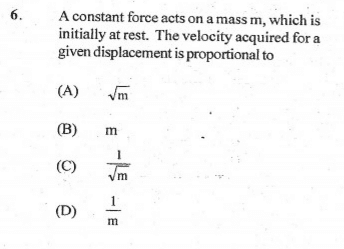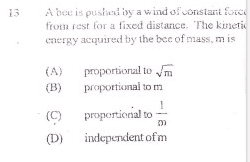# Relationship between Velocity, Kinetic Energy and Mass

## Homework Statementi) F=ma=mv/t
ii) E=mv2/2

## The Attempt at a Solution

Now based on equation 1 , I have concluded that the velocity gained is inversely proportional to m, and that so is the kinetic energy , I have seen otherwise.[/B]

BvU
Homework Helper
How can you conclude that if equation 1 does not contain any distance/displacement at alll ? You need other equations !

•Nile Anderson
How can you conclude that if equation 1 does not contain any distance/displacement at alll ? You need other equations !
Oh hmmmm, x=at^2/2, which means, a=2x/t^2, which means that mv/t=2mx/t^2=F, so does this mean they are independent , I am sorry , I am a little weak.Could you please elaborate?

BvU
Homework Helper
mv/t = F doesn't help you if you don't know how t depends on m.

Would the exercise be easier for you if the text would read:

You have a force ##F## to accelerate a mass ##m## from rest with constant acceleration over a distance ##d##. What is the final velocity ?​

•Nile Anderson
mv/t = F doesn't help you if you don't know how t depends on m.

Would the exercise be easier for you if the text would read:

You have a force ##F## to accelerate a mass ##m## from rest with constant acceleration over a distance ##d##. What is the final velocity ?​
I think I see something , the energy gained by the system would be Fd=mad, so this implies that mv^2/2=mad, v^2/2=ad, so this would mean the kinetic energy is independent of m ? Further a=F/m , v=sqrroot(Fd/m), does that mean v varies in an inverse proportion with sqrt(m)

mv/t = F doesn't help you if you don't know how t depends on m.

Would the exercise be easier for you if the text would read:

You have a force ##F## to accelerate a mass ##m## from rest with constant acceleration over a distance ##d##. What is the final velocity ?​
Sir ?

BvU
Homework Helper
kinetic energy is independent of m
Correct
Further a=F/m , v=sqrroot(Fd/m), does that mean v varies in an inverse proportion with sqrt(m)
Bingo.

Can you also do the alternative route ? ## \ d = {1\over 2} at^2\ ## tells you ##\ t \propto \sqrt d\ ## and you already had ##\ mv/t = F ##

Correct
Bingo.

Can you also do the alternative route ? ##\ d = {1\over 2} at^2} \ ## tells you ##\ t \propto \sqrt d\ ## and you already had ##\ mv/t = F ##
Thank you so much sir , I get it now

Correct
Bingo.

Can you also do the alternative route ? ## \ d = {1\over 2} at^2\ ## tells you ##\ t \propto \sqrt d\ ## and you already had ##\ mv/t = F ##
Hmmmmm

Right , I get back the same place sir, thank you so much sir , that helped alot.

BvU
Homework Helper
Hmmm as in "delicious" or Hmmm as in "I don't belive a word of what I read"?

Hmmm as in "delicious" or Hmmm as in "I don't belive a word of what I read"?
lol "hmmm" as in intriguing

BvU
$$\ d = {1\over 2} at^2\ \Rightarrow t = \sqrt{2d\over a} \Rightarrow v = at = {F\over m} \sqrt{2dm\over F} \Rightarrow v\propto 1/\sqrt m$$